Ch 5. Fundamental Laws (Differential) Multimedia Engineering Fluids ConservationMass Navier-Stokesand Euler's ConservationEnergy
 Chapter 1. Basics 2. Fluid Statics 3. Kinematics 4. Laws (Integral) 5. Laws (Diff.) 6. Modeling/Similitude 7. Inviscid 8. Viscous 9. External Flow 10. Open-Channel Appendix Basic Math Units Basic Equations Water/Air Tables Sections Search eBooks Dynamics Fluids Math Mechanics Statics Thermodynamics Author(s): Chean Chin Ngo Kurt Gramoll ©Kurt GramollFLUID MECHANICS - THEORY

The differential form of the linear momentum equation (also known as the Navier-Stokes equations) will be introduced in this section. Euler's equations for inviscid flow is also discussed.

Navier-Stokes Equations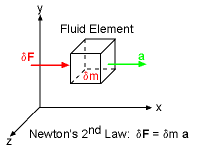Newton's Second Law

The integral form of the linear momentum equation was discussed in the Linear Momentum Integral Equation section. Recall, Newton's second law on a differential fluid element is

δF = δm a

where δF is the resultant force acting on the fluid element (mass = δm). a is the acceleration of the fluid element, and it is given by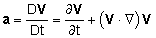Expanding into its Cartesian components yields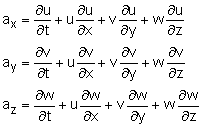There are two types of forces acting on the fluid element: body force (δFB) and surface force (δFS).

δF = δFB + δFS

The only body force considered here is the weight of the fluid element. That is,

δFB = δm g = δm (gx i + gy j + gz k)

Generally, gravity only acts in one direction, but since the coordinate system is not set, all three terms are included for the general case. Other body forces, such as those due to the magnetic and electric fields, can be fit into this framework, but they are not covered in this eBook.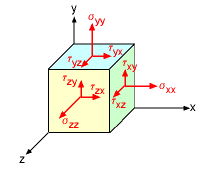Notations for the Stresses /p>Surface Forces in the x-direction

The surface forces are due to the stresses exerted on the sides of the fluid element. There are two types of stresses applied on the surface: normal stress (σij) and shear stress (τij). Normal stress acts perpendicular to the surface while shear stress is tangential to the surface. The subscript i refers to the axis normal to the surface, and the subscript j represents the direction of the stress. The notation of the stresses is illustrated further in the animation shown on the left.

All surface forces acting in the x-direction on the fluid element are shown in the figure. A summation of the surface forces in the x-direction yields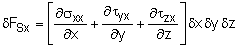Note that the stresses are multiplied by the area to obtain the surface forces. Similarly, the total surface forces in the y- and z-directions (not shown in figure) are obtained as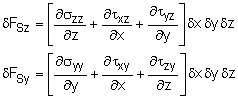The resultant surface force is then given as

δFS = δFSx i + δFSy j + δFSz k

The mass of the fluid element can be expressed in terms of its volume and fluid density (δm = ρ δxδyδz), so that the linear momentum equation in Cartesian coordinates reduces to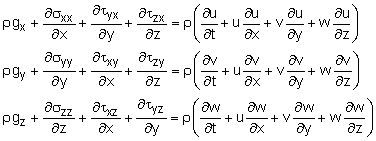For Newtonian fluids, such as water, oil and air, the shear stress field is symmetric, and it is related to the rate of shear strain in a linear fashion. The results are (derivation details not given here),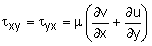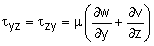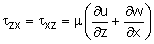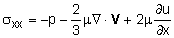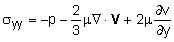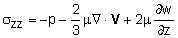where μ is the viscosity of the fluid. Notice, the pressure term, p, only acts normal to the surface for each element face. Also, the pressure is assumed to be the same on all three faces, i.e. hydrostatic pressure.

For incompressible flow, the term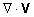is zero based on the continuity equation. The linear momentum equations thus become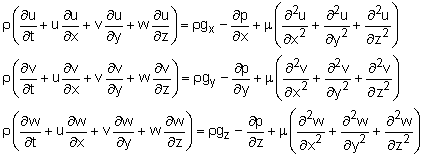The above equations are generally referred to as the Navier-Stokes equations, and commonly written as a single vector form,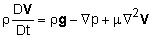Although the vector form looks simple, this equation is the core fluid mechanics equations and is an unsteady, nonlinear, 2nd order, partial differential equation. It is extremely hard to solve, and only simple 2D problems have been solved. Computational Fluid Dynamics (CFD) is most often used to solve the Navier-Stokes equations.

Euler's Equations

For inviscid flow (μ = 0), the Navier-Stokes equations reduce to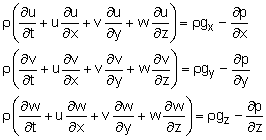The above equations are known as Euler's equations. Note that the equations governing inviscid flow have been simplified tremendously compared to the Navier-Stokes equations; however, they still cannot be solved analytically due to the complexity of the nonlinear terms (i.e., u ∂u/∂x, v ∂u/∂y, w ∂u/∂z, etc.). Hence, in the study of fluid mechanics, numerical methods such as the finite element and finite difference methods (along with the use of computers) are often used to approximate the fluid flow problems. Euler's equations can be written in vector form as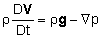Euler's equations can be simplified further to obtain Bernoulli's equation, which is applicable to steady, incompressible, inviscid flow along a streamline. A more detailed discussion on this subject is given in the solutions for the case study presented in this section.

Practice Homework and Test problems now available in the 'Eng Fluids' mobile app
Includes over 250 problems with complete detailed solutions.
Available now at the Google Play Store and Apple App Store.